# Learn Iteration using Loops in Python

0
4130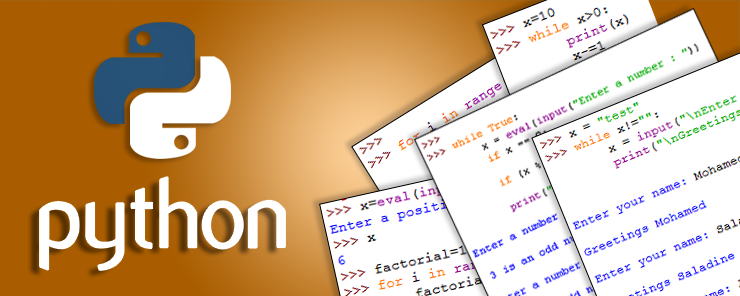In the previous article (Part 5 – Decision making using IF Conditions), I told you that life never goes one way. Actually, life in general goes in cycles; you wake up everyday at the same hour, wash your face, brush your teeth, take your breakfast, kiss your kids, and leave for work. You get to work at 8:00 o’clock, check your mail, reply urgent emails, check your calendar, have your coffee, perform your daily tasks, respond to new requests, take lunch break, attend the meeting scheduled a week ago, then leave at 4:00 P.M. The next weekday, you almost do the same things at the same hours. This is the daily cycle. Every week, has five working days, two days weekend. Again, this is the weekly cycle. I think you have already guessed the next cycles; the monthly cycle, and the yearly cycle. In computer programming, we use a different name to refer to such cycles: Loops.

Loops

A Loop is used to repeat the execution of a block of code (usually called the Loop Body).
The most commonly-used Loop types are: the for loop, and the while loop.

For Loop
The syntax of the for loop is:

```for var in range (start, end, step):
Statement 1
..
..
Statement n
```

Where:
var is the name of the loop variable,
range() is a built-in function that returns a sequence of numbers between start and end using a step value step (Default step = 1), and
Statements 1 through n are the loop body to be executed repeatedly.

Example
The following example prints the numbers from 1 to 20: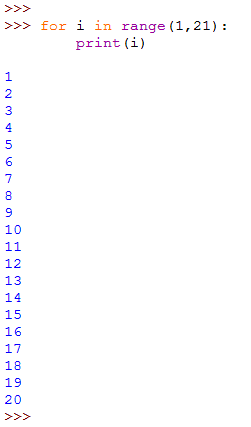Example

This script adds numbers from 1 to 10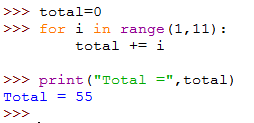Example

Consider a case if Python didn’t have a ready-made function to calculate the factorial of an integer math.factorial(). Now, your task as a developer is to write a script that calculates the factorial for an integer entered by the user. I know you are clever enough to do it, so please write it in your Python interactive shell and compare your code to this one:

By definition, the factorial of the integer x is the result of multiplying x by x-1 by x-2 …. by 2, by 1. So, a simple for loop that loops on the range 1 to x, and repeatedly multiplies the loop variable by the value stored in the variable factorial (initialized to 1) will give us at the end the factorial of x.

Notes:

• In the last two examples, notice the usage of the += and *= operators, to make accumulative addition and multiplication operations, respectively.

• Notice that for the loop variable to loop on the range 1 to x inclusively, we have to use the range() function with arguments 1, and x+1 (for the value x to be included).

* * * * * *

While Loop
The syntax of the while loop is:

```while condition:
Loop Body
```

While the for loop repeats execution for each value in range of values, the while loop continues to execute as long as the condition in the loop definition is met.

Example
This script prints the integers from 10 to 1 (in descending order).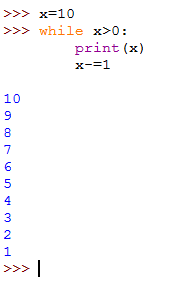The script initializes the variable x to the value 10. Then the while loop is defined to repeat as long as x is greater than 0. The loop body first prints the “current” value of x, then increments the value of x by 1.

Note:

If you forget to update the value of x (or if you update it the wrong way; i.e. by incrementing it), the loop will never stop execution. This case is known as infinite loop.

* * * * * *

I guess you know now how to re-write the scripts that add numbers from 1 to 11 and the one that calculates factorial for an integer using the while loop instead of the for loop. So, I will leave them for you as an exercise. Now, let’s concentrate on tasks that the while loop can achieve, while the for loop couldn’t.

Example

This script asks the user to enter a name, then prints that name with a greeting. This continues until the user enters an empty string.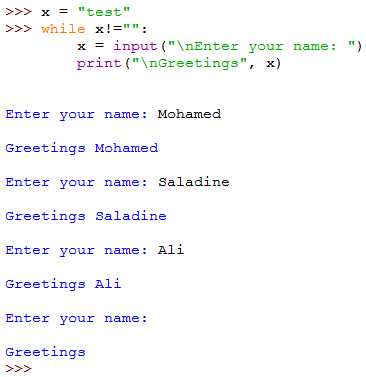First, the script initializes the variable x to a temporary string. This is essential for the script not to generate an error when the while loop checks the value of x for the first time.
Then, the while loop checks if the variable x is not equal to the empty string “”. If so, the loop body is executed:

• The script asks the user to enter a name.
• The user’s input is assigned to the variable x.
• The script prints the word “Greetings” followed by the name entered by the user.

* * * * * *

Continue and Break

There will be cases in which you would need to skip the execution of a specific iteration, or to escape the entire loop completely. For these cases, two commands are used; they are continue and break, respectively.

Consider the following script that asks the user to enter a number. The script will check and tell if the input is an odd number. If not, nothing is printed. This will be repeated until the user enters 0.

>while True

Sounds strange Doesn’t it?!

Actually, there is nothing strange at all. You remember when I told you (several paragraphs ago) that the syntax of the while loop is

```while condition:
Loop Body
```

Again, remind me: what the condition is! It is an expression that evaluates to either True or False.

And what did I do?! I simply put a True value after the while statement, which is equivalent to saying: keep executing the following loop body as long as the condition is True (which is always the case because the condition used is just the literal value True). This is called an infinite (endless) loop.

• An if statement checks if x equals 0, which the loop exit condition. If so, the break statement is executed that breaks (exits) the loop transferring the control to the next line after the loop (if any).

• The expression (x % 2) divides x by 2 and returns the reminder. We know from arithmetic that even numbers divide by two without any reminder. So, if the reminder equals 0, then x is an even number. In that case, we don’t want to execute anything, so the continue statement just skips the rest of the loop body and jumps to the next iteration.

• If x is neither a 0 nor an even number, it must be an odd number. So, the print() function tells the user that the number he has just entered is an odd number. No need here for further checks.

* * * * * *

This is how loops work. A simple, yet very important topic.